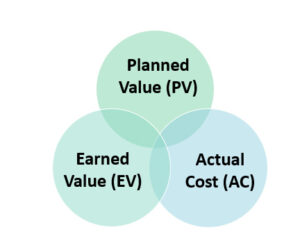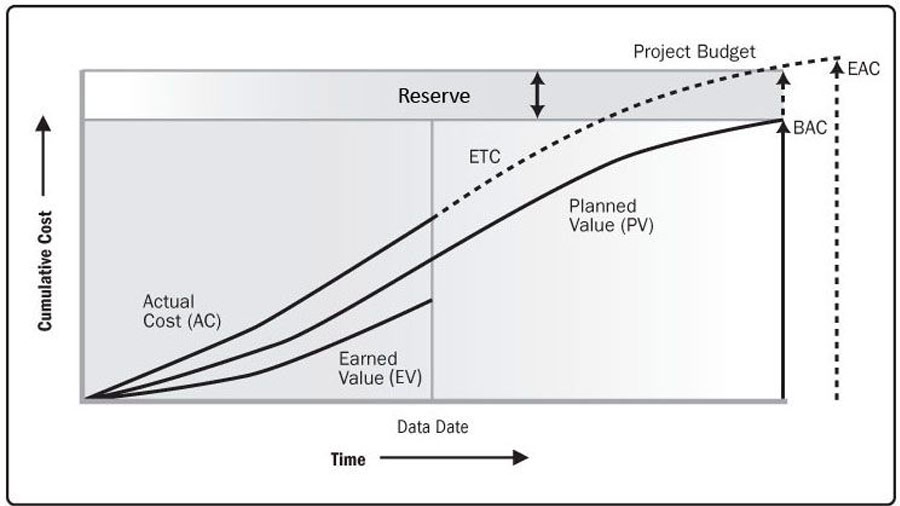Earned value management template is all about managing the budget of the project and evaluate the overall performance of the project. EVM templates allows you to see your cost, scope and schedule in one baseline. It actually measures the progress of the project in accordance with the cost.

Let us take an example to understand the earned value analysis template in better way. Suppose we want to pain t our home. We estimated 20 days for the composition of work and the estimated cost is 2000rs.

## What is Earned Value analysis Template? Examples & Benefits

We started the work. After 10 days, let’s say the cost spend is \$8(less than the estimated price) and 40% work has accomplished (required task was 50%). We should have complete 50% work at the cost of \$10.

Now in order to access our project, by comparing the actual cost with the planned one will not work for us. As the actual cost is less than the planned that means we are under budget.

But the complete work is less than the estimated one that means we are not on the schedule. So in order to compare the progress of the project with the plan we need earned value analysis template, earned value (40%*2000) =80

1. EVM is more helpful than a project tracking template
2. It answers all the questions relating to your project so accurately
3. Estimates the time for the completion of your project
4. It defines you the current situation of your project as well as the progress of the project
5. This is helpful in reaching the project timeline easily
6. This template gives better project visibility and accountability
7. It also provides the clear communication of all the activities involved in a project

Excel provides verity of template that very user friendly. it helps in the project management the EVM is another such type pf template provided by excel that is helpful in improving our business skills.

### Key Futures Of Earned Value Management Template

For the better understanding of the principal of the template it is necessary to understand the basic terms involved#### Planned Value (PV)

It is termed as the budgeted cost of work scheduled (BCWS). When you start any project you estimate the cost of every task of the project. For the long term project this cost is divided into periods and EVM gives you the budgeted cost for each period. This will serve as a base for the comparison.

#### Actual Cost (AC)

The actual cost of work performed (ACWP) includes all the money you have spent on different portions of your task including the labor and the material. It alone cannot give the result but still it is an important term to consider.

#### Earned Value (EV)

This the budgeted cost of work performed (BCWP).OR the amount of work completed.in the earned value management template it is calculated by multiplying the percentage work completed with the total budget cost of the project.

## How To Conduct Earned Value Analysis Through Template

Earned value of your project can be calculated using the template, for the effective Analysis of earned value following should be priorities

• Find out the percentage of completion of each task
• Calculate the planned value (PV)
• Calculate the earned value (EV)
• Determine the actual cost (AC)
• Calculation of cost variance (CV)
• Calculate schedule variance (SV)
• Calculation other effective indicators ( SPI, CPI,EAC, ETC and TCPI )
• Finally conclude the results

In order to find out above indicators specific formulas are use which are as follows

### Cost Variance

It shows the deviations from your planned budget. If the value is negative that means you are spending more than planned and if the value is positive that means you are under budget.

Cost Variance (CV) = Earned Value (EV) – Actual Value (EV)

### Schedule Variance

It shows the deviations from the schedule. If the value is negative that means you are not on schedule and if the value is positive that means you are ahead of schedule.

Schedule Variance (SV) = Earned Value (EV) – Planned Value (PV)

### Cost Performance Index

Cost Performance Index (CPI) = Earned Value (EV)/Actual Cost (AC)

### Schedule Performance Index

Schedule Performance Index (SPI) = Earned Value (EV)/Planned Value (PV)

## Estimate At Completion Template (EVM)

Estimate at completion actually estimates the budget of the project while the project is in progress. It makes correspondence with the budget at completion (BAC) which involves the estimation of total cost in the planning phase.In budget at completion (BAC) there are only assumptions involved, before calculation of EAC some further implementations must be made for the future of the project and that includes

• Make an estimate to complete the assumption you have made
• Make sure project is completing within budget rate
• The performance in the future will be in accordance with the cost performance index and cost variance
• The performance of future will be in accordance with the cost performance index and schedule performance index.

### Use Of Estimate At Completion Formulas

EAC formulas are different according to the situation and this article helps you to find out which EAC formula is use in which condition

• If the initial estimations were not correct and need to be ignored or the initial estimation is not forecasting an accurate result then the formula use is

EAC =AC +ETC

(Estimate at completion equals actual cost plus estimate at completion)

• When there is no considerable deviation from the actual estimate, Use the following formula

EAC = BAC/CPI

(Estimate at completion equals budget at completion divided by cost performance index)

• When the project is facing some issues related to time but the budget performance is accurate according to the plan, use the following formula

EAC = AC + (BAC – EV)

(Estimate at completion equals actual cost plus budget at completion minus earned value)

• To reach at the updated budget forecast keeping in account the cost and schedule, this formula is use

EAC =AC + (BAC – EV)/SPI + CPI

(Estimate at completion equals actual cost plus budget at completion minus earned value divided by schedule performance index plus cost performance index)

EAC is an independent forecast in the project management and it will cost to accomplish the tasks in work breakdown structure (WBS). This is very helpful for the manager in managing the project in a very effective way.For more information about Earned Value Analysis Template excel, you can contact PMITOOLS.NET

References : Earned Value Management